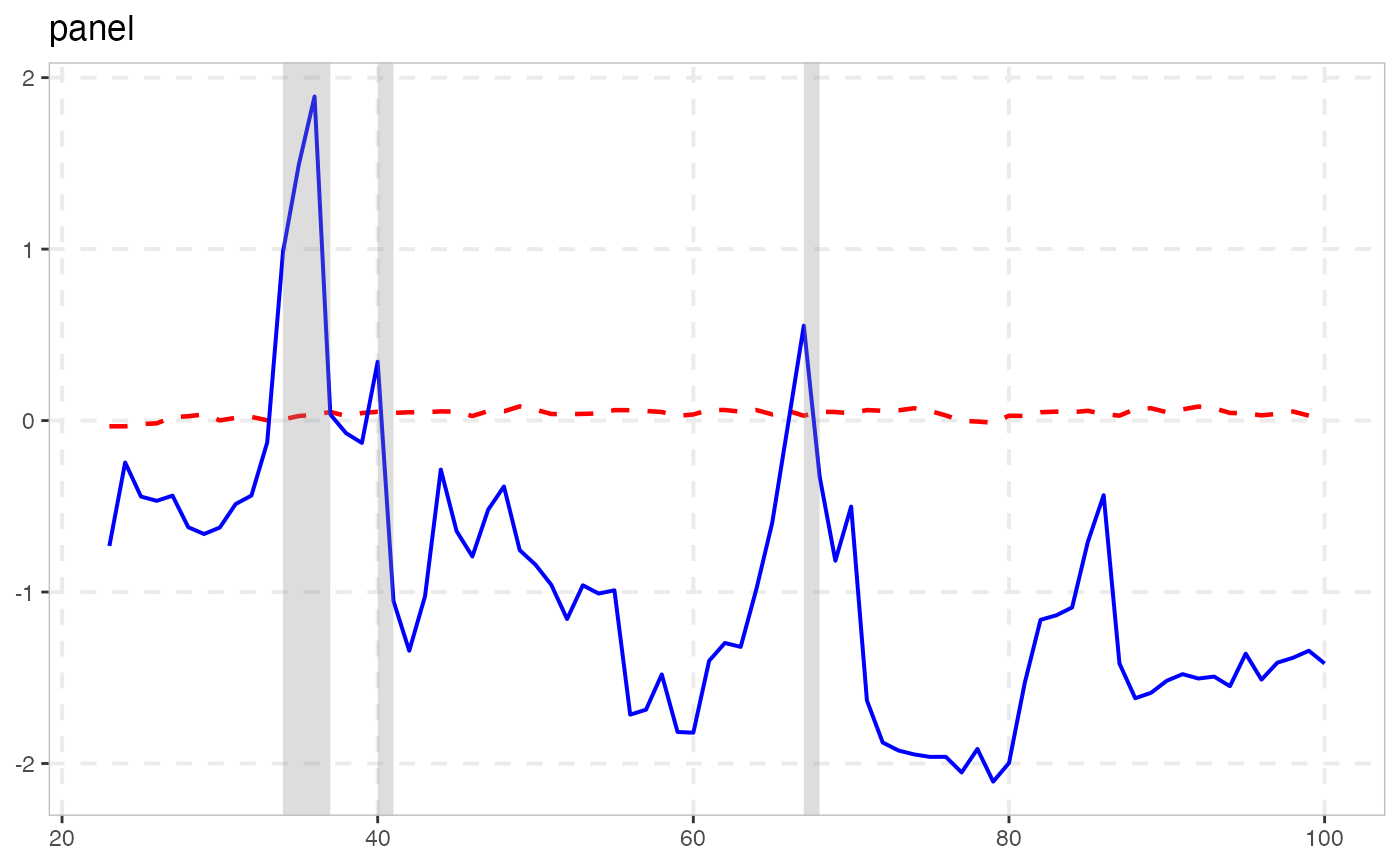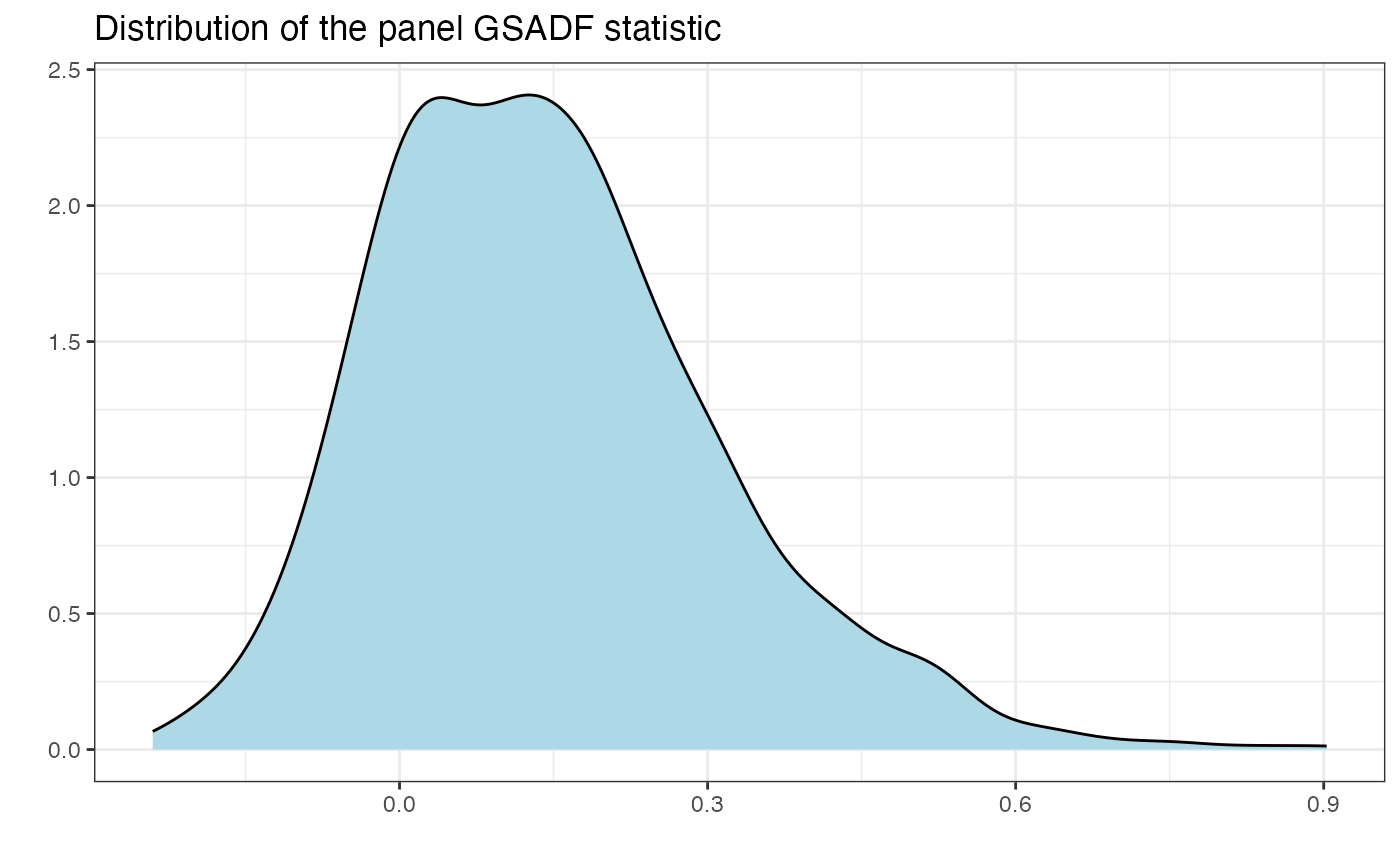radf_sb_cv computes critical values for the panel recursive unit root test using the sieve bootstrap procedure outlined in Pavlidis et al. (2016). radf_sb_distr computes the distribution.

radf_sb_cv(data, minw = NULL, lag = 0L, nboot = 500L, seed = NULL)

radf_sb_distr(data, minw = NULL, lag = 0L, nboot = 500L, seed = NULL)

## Arguments

data

A univariate or multivariate numeric time series object, a numeric vector or matrix, or a data.frame. The object should not have any NA values.

minw

A positive integer. The minimum window size (default = $$(0.01 + 1.8/\sqrt(T))T$$, where T denotes the sample size).

lag

A non-negative integer. The lag length of the Augmented Dickey-Fuller regression (default = 0L).

nboot

A positive integer. Number of bootstraps (default = 500L).

seed

An object specifying if and how the random number generator (rng) should be initialized. Either NULL or an integer will be used in a call to set.seed before simulation. If set, the value is saved as "seed" attribute of the returned value. The default, NULL, will not change rng state, and return .Random.seed as the "seed" attribute. Results are different between the parallel and non-parallel option, even if they have the same seed.

## Value

For radf_sb_cv A list A list that contains the critical values for the panel BSADF and panel GSADF test statistics. For radf_wb_dist a numeric vector that contains the distribution of the panel GSADF statistic.

radf_mc_cv for Monte Carlo critical values and radf_wb_cv for wild Bootstrap critical values

## Examples

# \donttest{

rsim_data <- radf(sim_data, lag = 1)

# Critical vales should have the same lag length with \code{radf()}
sb <- radf_sb_cv(sim_data, lag = 1)

tidy(sb)
#> # A tibble: 3 × 3
#>   <fct> <fct>       <dbl>
#> 1 panel 90          0.356
#> 2 panel 95          0.451
#> 3 panel 99          0.637

summary(rsim_data, cv = sb)
#>
#> ── Summary (minw = 19, lag = 1) ─────────────── Sieve Bootstrap (nboot = 500) ──
#>
#> panel :
#> # A tibble: 1 × 5
#>   stat        tstat  90  95  99
#>   <fct>       <dbl> <dbl> <dbl> <dbl>
#> 1 gsadf_panel  1.89 0.356 0.451 0.637
#>

autoplot(rsim_data, cv = sb)# Simulate distribution
sdist <- radf_sb_distr(sim_data, lag = 1, nboot = 1000)

autoplot(sdist)# }